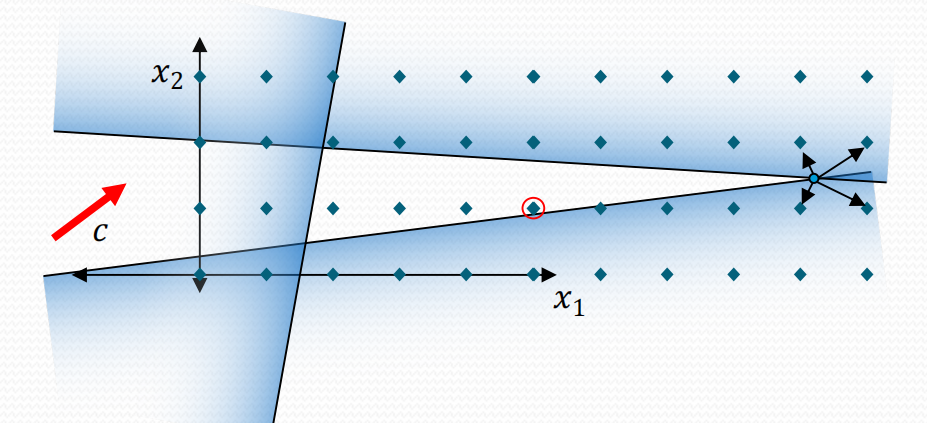# Python小白的数学建模课-04.整数规划

『Python小白的数学建模课 @ Youcans』带你从数模小白成为国赛达人。

## 1. 从线性规划到整数规划

### 1.1 为什么会有整数规划？

• 完全整数规划，全部变量都要求是整数；
• 混合整数规划，部分变量要求是整数；
• 0-1整数规划，变量的取值只能是 0 或 1；
• 混合0-1规划，部分变量的取值只能是 0 或 1。

0-1整数规划 是非常重要也非常特殊的整数规划，需要在另外的文章进行讨论。

### 1.2 四舍五入就能得到整数解吗？## 3. PuLP 求解整数规划问题

### 3.2 建模过程分析

#### 3.2.1 问题定义

1. 决策变量是问题中可以在一定范围内进行变化而获得不同结果的变量。

对于问题 1，问题描述中说的很明确，希望通过改变甲、乙两种饮料的产量使总利润最大，甲、乙两种饮料的产量就是决策变量。

对于问题 2 则要注意，如果只看前一句，就是比较问题 1 与问题 2 的利润，还是把甲、乙两种饮料的产量作为决策变量。但要回答后一句“投资多少合理”，这就出现了一个新的变量“投资额”，因此对问题 2 要建立 3个决策变量：甲产量、乙产量和投资额。

2. 目标函数是决策变量的函数，我们希望通过改变决策变量的值而获得目标函数的最大值或最小值，通常是总成本（最小）、总利润（最大）、总时间（最短）。

对于本案例，每个问题都是希望获得最大利润，目标函数都是总利润，问题是求目标函数即总利润的最大值。

3. 约束条件是决策变量所要满足的限制条件。

约束条件 3 种情况：
一是不等式约束，例如题目指出共有原料 60千克、工人 150名，因此生产计划所用的原料、工人的需求不能大于题目中数值。
二是等式约束，本题没有等式约束条件。
三是决策变量取值范围的约束。
通常，题目隐含着决策变量大于等于 0 的条件，例如工人人数、原料数量都要大于等于 0。
另外，如果能通过分析前面的等式约束或不等式约束，得出决策变量的上限，将会极大的提高问题求解的速度和性能。后文将对此举例说明。

#### 3.2.2 模型构建

$max\;f(x) = 10*x_1 + 9*x_2\\ s.t.:\begin{cases} 6*x_1 + 5*x_2 \leq 60\\ 10*x_1 + 20*x_2 \leq 150\\ 0 \leq x_1 \leq 8\\ x_2 \geq 0 \end{cases}$

$x_1 \leq 15\\ x_2 \leq 7.5$

$max\;f(x) = 10*x_1 + 9*x_2 - x_3\\ s.t.:\begin{cases} 6*x_1 + 5*x_2 \leq 60 + x_3/0.8\\ 10*x_1 + 20*x_2 \leq 150\\ 0 \leq x_1 \leq 15\\ 0 \leq x_2 \leq 7.5\\ x_3 \geq 0 \end{cases}$

#### 3.2.3 模型求解

（0）导入 PuLP库函数

    import pulp


（1）定义一个规划问题

    ProbLP1 = pulp.LpProblem("ProbLP1", sense=pulp.LpMaximize)    # 定义问题 1，求最大值


pulp.LpProblem 用来定义问题的构造函数。"ProbLP1"是用户定义的问题名。

（2）定义决策变量

    x1 = pulp.LpVariable('x1', lowBound=0, upBound=15, cat='Continuous')  # 定义 x1
x2 = pulp.LpVariable('x2', lowBound=0, upBound=7.5, cat='Continuous')  # 定义 x2


pulp.LpVariable 用来定义决策变量的函数。'x1'、'x2' 是用户定义的变量名。

   x1 = pulp.LpVariable('x1', lowBound=0, upBound=15, cat='Integer')  # 定义 x1，变量类型：整数
x2 = pulp.LpVariable('x2', lowBound=0, upBound=7.5, cat='Integer')  # 定义 x2，变量类型：整数


（3）添加目标函数

    ProbLP1 += (10*x1 + 9*x2)  # 设置目标函数 f(x)


（4）添加约束条件

    ProbLP1 += (6*x1 + 5*x2 <= 60)  # 不等式约束
ProbLP1 += (10*x1 + 20*x2 <= 150)  # 不等式约束


添加约束条件使用 "问题名 += 约束条件表达式" 格式。
约束条件可以是等式约束或不等式约束，不等式约束可以是 小于等于 或 大于等于，分别使用关键字">="、"<="和"=="。

（5）求解

    ProbLP1.solve()
print(ProbLP1.name)  # 输出求解状态
print("Status:", pulp.LpStatus[ProbLP1.status])  # 输出求解状态
for v in ProbLP1.variables():
print(v.name, "=", v.varValue)  # 输出每个变量的最优值
print("F1(x) =", pulp.value(ProbLP1.objective))  # 输出最优解的目标函数值


solve() 是求解函数，可以对求解器、求解精度进行设置。
PuLP默认采用 CBC 求解器来求解优化问题，也可以调用其它的优化器来求解，但需要另外安装。

### 3.3 Python 例程

# mathmodel05_v1.py
# Demo05 of mathematical modeling algorithm
# Solving integer programming with PuLP.
# Crated：2021-05-31
# Python小白的数学建模课 @ Youcans

import pulp      # 导入 pulp 库

# 主程序
def main():

# 模型参数设置
"""
问题描述：
某厂生产甲乙两种饮料，每百箱甲饮料需用原料6千克、工人10名，获利10万元；每百箱乙饮料需用原料5千克、工人20名，获利9万元。
今工厂共有原料60千克、工人150名，又由于其他条件所限甲饮料产量不超过8百箱。
（1）问如何安排生产计划，即两种饮料各生产多少使获利最大？
（2）若投资0.8万元可增加原料1千克，是否应作这项投资？投资多少合理？
（3）若不允许散箱（按整百箱生产），如何安排生产计划，即两种饮料各生产多少使获利最大？
（4）若不允许散箱（按整百箱生产），若投资0.8万元可增加原料1千克，是否应作这项投资？投资多少合理？
"""

# 问题 1：
"""
问题建模：
决策变量：
x1：甲饮料产量（单位：百箱）
x2：乙饮料产量（单位：百箱）
目标函数：
max fx = 10*x1 + 9*x2
约束条件：
6*x1 + 5*x2 <= 60
10*x1 + 20*x2 <= 150
x1, x2 >= 0，x1 <= 8
此外，由 x1,x2>=0 和 10*x1+20*x2<=150 可知 0<=x2<=7.5
"""
ProbLP1 = pulp.LpProblem("ProbLP1", sense=pulp.LpMaximize)    # 定义问题 1，求最大值
x1 = pulp.LpVariable('x1', lowBound=0, upBound=8, cat='Continuous')  # 定义 x1
x2 = pulp.LpVariable('x2', lowBound=0, upBound=7.5, cat='Continuous')  # 定义 x2
ProbLP1 += (10*x1 + 9*x2)  # 设置目标函数 f(x)
ProbLP1 += (6*x1 + 5*x2 <= 60)  # 不等式约束
ProbLP1 += (10*x1 + 20*x2 <= 150)  # 不等式约束
ProbLP1.solve()
print(ProbLP1.name)  # 输出求解状态
print("Status youcans:", pulp.LpStatus[ProbLP1.status])  # 输出求解状态
for v in ProbLP1.variables():
print(v.name, "=", v.varValue)  # 输出每个变量的最优值
print("F1(x) =", pulp.value(ProbLP1.objective))  # 输出最优解的目标函数值

# 问题 2：
"""
问题建模：
决策变量：
x1：甲饮料产量（单位：百箱）
x2：乙饮料产量（单位：百箱）
x3：增加投资（单位：万元）
目标函数：
max fx = 10*x1 + 9*x2 - x3
约束条件：
6*x1 + 5*x2 <= 60 + x3/0.8
10*x1 + 20*x2 <= 150
x1, x2, x3 >= 0，x1 <= 8
此外，由 x1,x2>=0 和 10*x1+20*x2<=150 可知 0<=x2<=7.5
"""
ProbLP2 = pulp.LpProblem("ProbLP2", sense=pulp.LpMaximize)    # 定义问题 2，求最大值
x1 = pulp.LpVariable('x1', lowBound=0, upBound=8, cat='Continuous')  # 定义 x1
x2 = pulp.LpVariable('x2', lowBound=0, upBound=7.5, cat='Continuous')  # 定义 x2
x3 = pulp.LpVariable('x3', lowBound=0, cat='Continuous')  # 定义 x3
ProbLP2 += (10*x1 + 9*x2 - x3)  # 设置目标函数 f(x)
ProbLP2 += (6*x1 + 5*x2 - 1.25*x3 <= 60)  # 不等式约束
ProbLP2 += (10*x1 + 20*x2 <= 150)  # 不等式约束
ProbLP2.solve()
print(ProbLP2.name)  # 输出求解状态
print("Status  youcans:", pulp.LpStatus[ProbLP2.status])  # 输出求解状态
for v in ProbLP2.variables():
print(v.name, "=", v.varValue)  # 输出每个变量的最优值
print("F2(x) =", pulp.value(ProbLP2.objective))  # 输出最优解的目标函数值

# 问题 3：整数规划问题
"""
问题建模：
决策变量：
x1：甲饮料产量，正整数（单位：百箱）
x2：乙饮料产量，正整数（单位：百箱）
目标函数：
max fx = 10*x1 + 9*x2
约束条件：
6*x1 + 5*x2 <= 60
10*x1 + 20*x2 <= 150
x1, x2 >= 0，x1 <= 8，x1, x2 为整数
此外，由 x1,x2>=0 和 10*x1+20*x2<=150 可知 0<=x2<=7.5
"""
ProbLP3 = pulp.LpProblem("ProbLP3", sense=pulp.LpMaximize)  # 定义问题 3，求最大值
print(ProbLP3.name)  # 输出求解状态
x1 = pulp.LpVariable('x1', lowBound=0, upBound=8, cat='Integer')  # 定义 x1，变量类型：整数
x2 = pulp.LpVariable('x2', lowBound=0, upBound=7.5, cat='Integer')  # 定义 x2，变量类型：整数
ProbLP3 += (10 * x1 + 9 * x2)  # 设置目标函数 f(x)
ProbLP3 += (6 * x1 + 5 * x2 <= 60)  # 不等式约束
ProbLP3 += (10 * x1 + 20 * x2 <= 150)  # 不等式约束
ProbLP3.solve()
print("Shan Status:", pulp.LpStatus[ProbLP3.status])  # 输出求解状态
for v in ProbLP3.variables():
print(v.name, "=", v.varValue)  # 输出每个变量的最优值
print("F3(x) =", pulp.value(ProbLP3.objective))  # 输出最优解的目标函数值

# 问题 4：
"""
问题建模：
决策变量：
x1：甲饮料产量，正整数（单位：百箱）
x2：乙饮料产量，正整数（单位：百箱）
x3：增加投资（单位：万元）
目标函数：
max fx = 10*x1 + 9*x2 - x3
约束条件：
6*x1 + 5*x2 <= 60 + x3/0.8
10*x1 + 20*x2 <= 150
x1, x2, x3 >= 0，x1 <= 8，x1, x2 为整数
此外，由 x1,x2>=0 和 10*x1+20*x2<=150 可知 0<=x2<=7.5
"""
ProbLP4 = pulp.LpProblem("ProbLP4", sense=pulp.LpMaximize)  # 定义问题 4，求最大值
print(ProbLP4.name)  # 输出求解状态
x1 = pulp.LpVariable('x1', lowBound=0, upBound=8, cat='Integer')  # 定义 x1，变量类型：整数
x2 = pulp.LpVariable('x2', lowBound=0, upBound=7, cat='Integer')  # 定义 x2，变量类型：整数
x3 = pulp.LpVariable('x3', lowBound=0, cat='Continuous')  # 定义 x3
ProbLP4 += (10*x1 + 9*x2 - x3)  # 设置目标函数 f(x)
ProbLP4 += (6*x1 + 5*x2 - 1.25*x3 <= 60)  # 不等式约束
ProbLP4 += (10*x1 + 20*x2 <= 150)  # 不等式约束
ProbLP4.solve()
print("Shan Status:", pulp.LpStatus[ProbLP4.status])  # 输出求解状态
for v in ProbLP4.variables():
print(v.name, "=", v.varValue)  # 输出每个变量的最优值
print("F4(x) =", pulp.value(ProbLP4.objective))  # 输出最优解的目标函数值

return

if __name__ == '__main__':  # Copyright 2021 YouCans, XUPT
main()  # Python小白的数学建模课 @ Youcans


### 3.4 Python 例程运行结果

Welcome to the CBC MILP Solver
Version: 2.9.0
Build Date: Feb 12 2015

ProbLP1
Status: Optimal
x1 = 6.4285714
x2 = 4.2857143
F1(x) = 102.8571427

ProbLP2
Status: Optimal
x1 = 8.0
x2 = 3.5
x3 = 4.4
F2(x) = 107.1

ProbLP3
Result - Optimal solution found
Status Shan: Optimal
Status: Optimal
x1 = 8.0
x2 = 2.0
F3(x) = 98.0

ProbLP4
Result - Optimal solution found
Status: Optimal
x1 = 8.0
x2 = 3.0
x3 = 2.4
F4(x) = 104.6


【本节完】